# The relationship between temperature and volume How Volume

• Slides: 18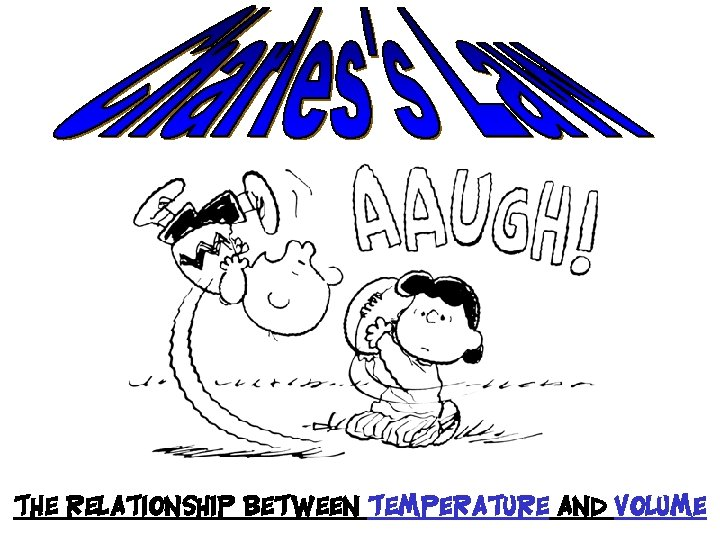The relationship between temperature and volume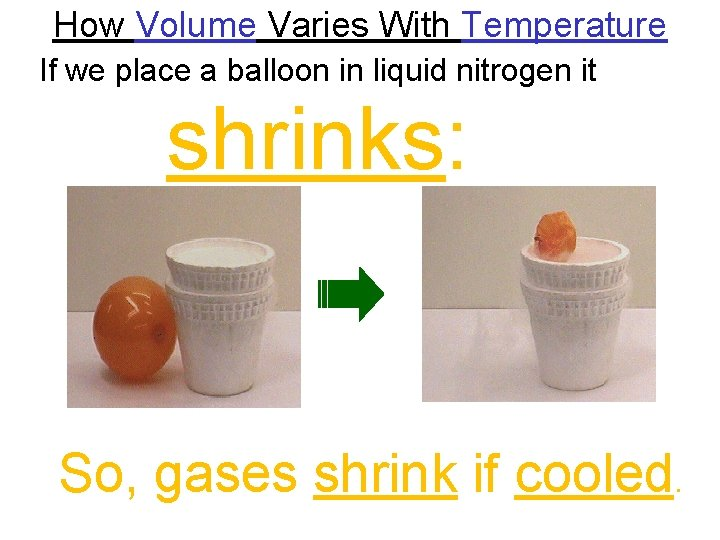How Volume Varies With Temperature If we place a balloon in liquid nitrogen it shrinks: So, gases shrink if cooled.How Volume Varies With Temperature Conversely, if we heat a gas it expands (as in a hot air balloon).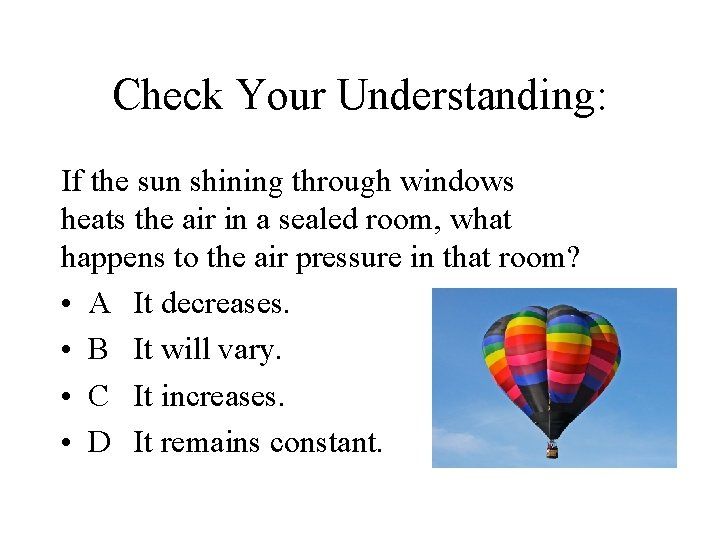Check Your Understanding: If the sun shining through windows heats the air in a sealed room, what happens to the air pressure in that room? • A It decreases. • B It will vary. • C It increases. • D It remains constant.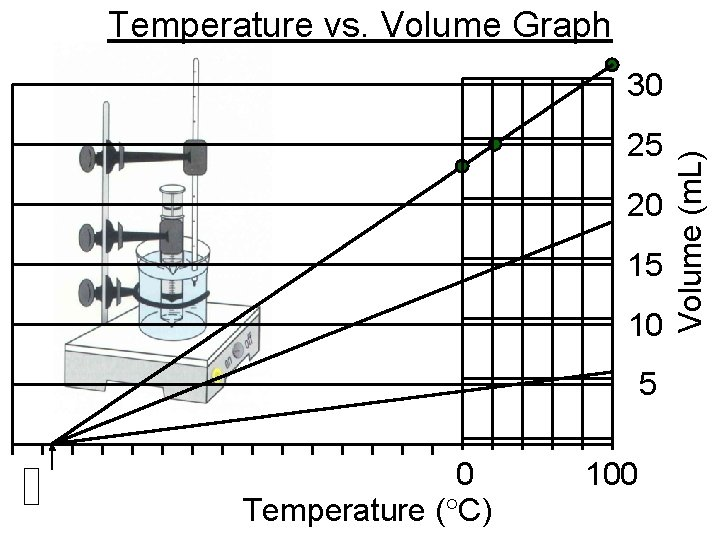Temperature vs. Volume Graph 25 20 15 10 5 0 Temperature ( C) 100 Volume (m. L) 30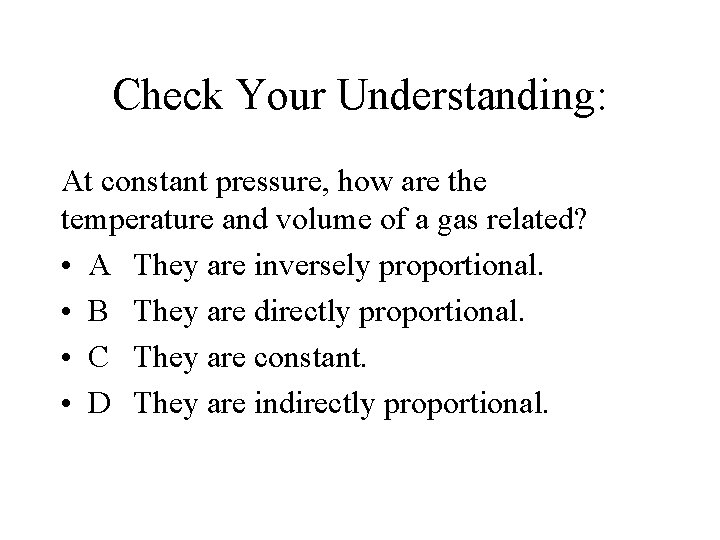Check Your Understanding: At constant pressure, how are the temperature and volume of a gas related? • A They are inversely proportional. • B They are directly proportional. • C They are constant. • D They are indirectly proportional.Charles’s Law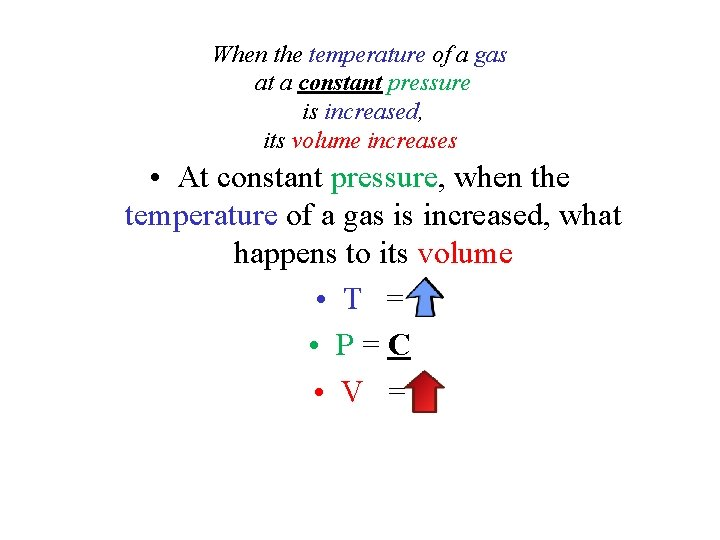When the temperature of a gas at a constant pressure is increased, its volume increases • At constant pressure, when the temperature of a gas is increased, what happens to its volume • T = • P=C • V =When the temperature of a gas at a constant pressure is decreased, its volume decreases • At constant pressure, when the temperature of a gas is decreased, what happens to its volume • T = • P=C • V =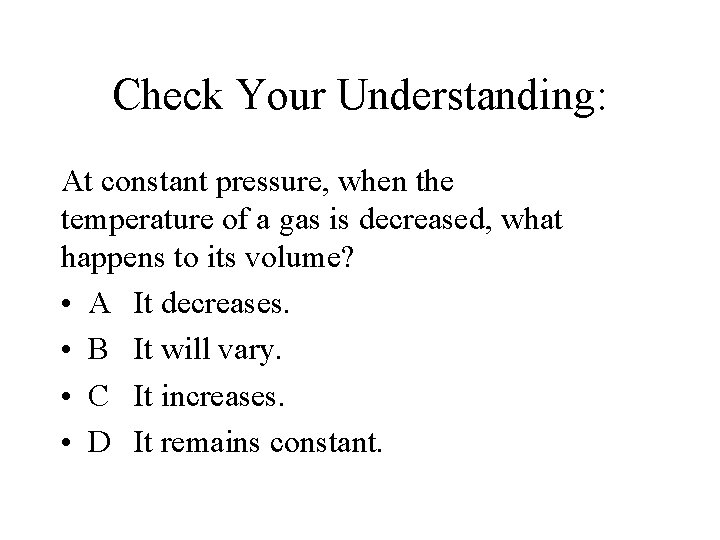Check Your Understanding: At constant pressure, when the temperature of a gas is decreased, what happens to its volume? • A It decreases. • B It will vary. • C It increases. • D It remains constant.bo. Yle’s law the relationship between pressure and volume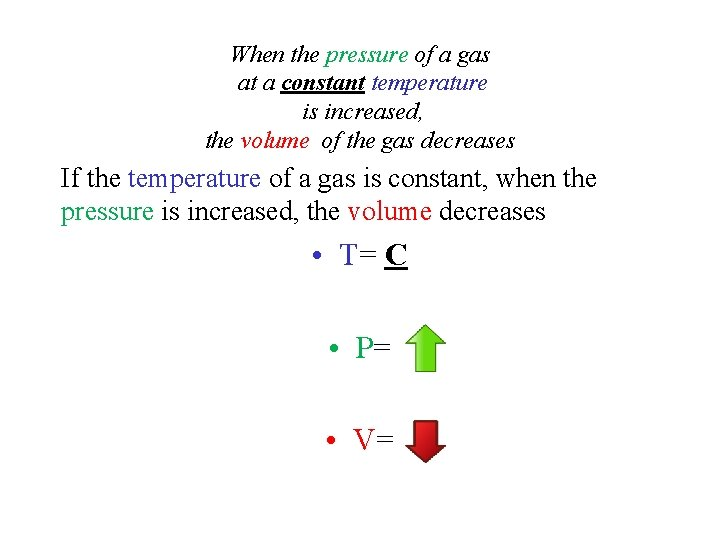When the pressure of a gas at a constant temperature is increased, the volume of the gas decreases If the temperature of a gas is constant, when the pressure is increased, the volume decreases • T= C • P= • V=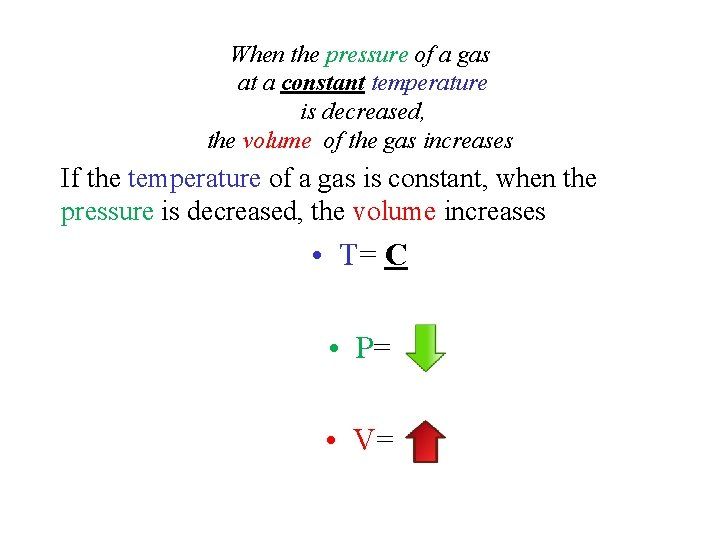When the pressure of a gas at a constant temperature is decreased, the volume of the gas increases If the temperature of a gas is constant, when the pressure is decreased, the volume increases • T= C • P= • V=T and P pg 65 no name…lets call it Sheffer’s Law T= P= V =C Directly Proportional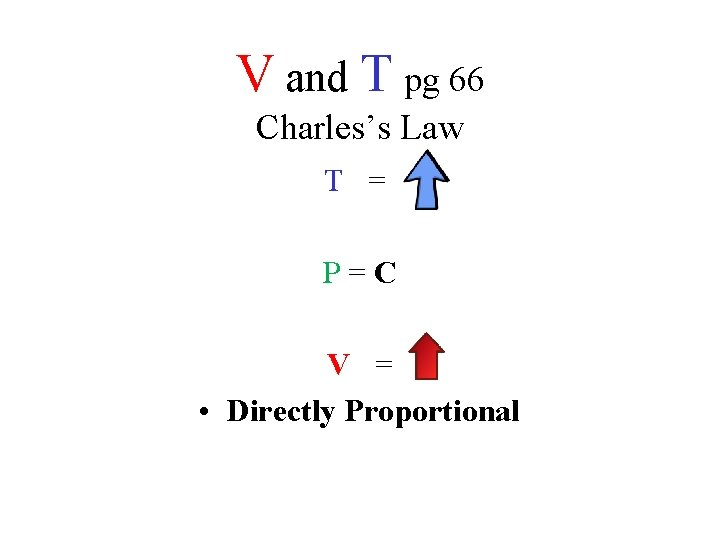V and T pg 66 Charles’s Law T = P=C V = • Directly Proportional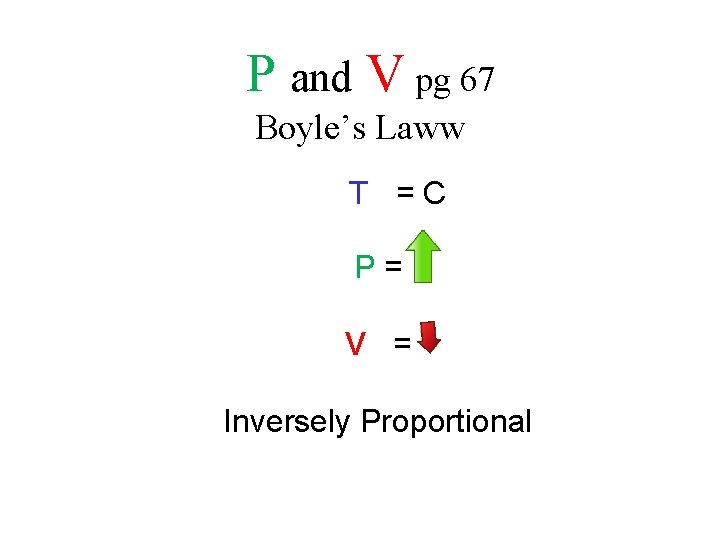P and V pg 67 Boyle’s Laww T =C P= V = Inversely ProportionalSolids, Liquids, and Gases Chapter 2 Study Guide A 1. 2. 3. 4. 5. 6. B C A D C B 7. C 8. C 9. B 10. C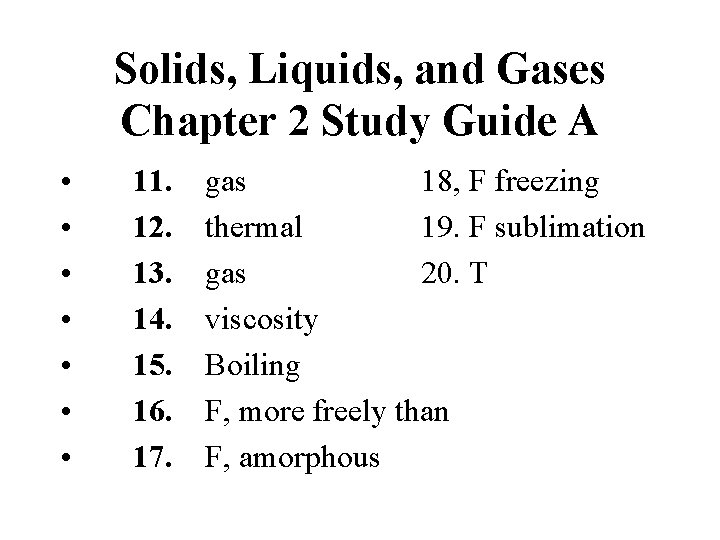Solids, Liquids, and Gases Chapter 2 Study Guide A • • 11. 12. 13. 14. 15. 16. 17. gas 18, F freezing thermal 19. F sublimation gas 20. T viscosity Boiling F, more freely than F, amorphous